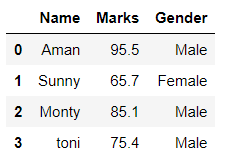Related Articles
Difference between Pandas VS NumPy
• Last Updated : 24 Oct, 2020

Pandas: It is an open-source, BSD-licensed library written in Python Language. Pandas provide high performance, fast, easy to use data structures and data analysis tools for manipulating numeric data and time series. Pandas is built on the numpy library and written in languages like Python, Cython, and C. In pandas, we can import data from various file formats like JSON, SQL, Microsoft Excel, etc.

Example:

## Python3

 `# Importing pandas library``import` `pandas as pd`` ` `# Creating and initializing a nested list``age ``=` `[[``'Aman'``, ``95.5``, ``"Male"``], [``'Sunny'``, ``65.7``, ``"Female"``],``       ``[``'Monty'``, ``85.1``, ``"Male"``], [``'toni'``, ``75.4``, ``"Male"``]]`` ` `# Creating a pandas dataframe``df ``=` `pd.DataFrame(age, columns``=``[``'Name'``, ``'Marks'``, ``'Gender'``])`` ` `# Printing dataframe``df`

Output:Numpy: It is the fundamental library of python, used to perform scientific computing. It provides high-performance multidimensional arrays and tools to deal with them. A numpy array is a grid of values (of the same type) that are indexed by a tuple of positive integers, numpy arrays are fast, easy to understand, and give users the right to perform calculations across arrays.

Example:

## Python3

 `# Importing Numpy package``import` `numpy as np`` ` `# Creating a 3-D numpy array using np.array()``org_array ``=` `np.array([[``23``, ``46``, ``85``],``                      ``[``43``, ``56``, ``99``],``                      ``[``11``, ``34``, ``55``]])`` ` `# Printing the Numpy array``print``(org_array)`

Output:

```[[23 46 85]
[43 56 99]
[11 34 55]]
```

Table of Difference Between Pandas VS NumPy

Attention geek! Strengthen your foundations with the Python Programming Foundation Course and learn the basics.

To begin with, your interview preparations Enhance your Data Structures concepts with the Python DS Course.

My Personal Notes arrow_drop_up